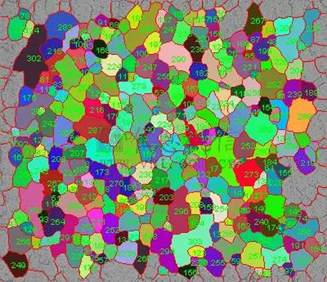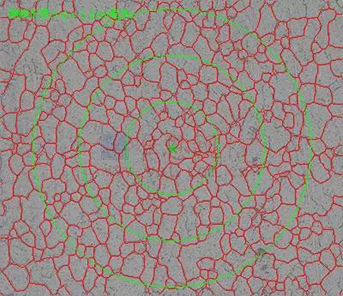# Spot Segregation: Basic Method for Determining Average Grain Size

30/03/2022
In general, there are three basic methods for determining the average grain size: the comparison method, the area method, and the intercept point method.Details as follows:
Comparison method: the comparison method does not need to calculate the grain and intercept. Compared with the standard series rating chart, there is generally a certain deviation (±0.5 grade) when evaluating the grain size by the comparison method. The reproducibility and reproducibility of the evaluation values are usually ±1 grade.
Area method: the area method is to calculate the number of grains in known area, and use the number of grains per unit area to determine the number of grain size grades. The accuracy of the method is a function of the calculated grain size, with an accuracy of the order of ±0.25 achievable with reasonable counts. The measurement results of the area method are unbiased, and the reproducibility is less than ±0.5 grades. The key to the grain size of the area method lies in the number of grains clearly divided by the grain interface.

Intersection point method: the number of intercept points is to calculate the number of intercept points of the intersection of the test line segment (or grid) of known length and the grain interface, and the number of intercept points per unit length is used to determine the number of grain size grades. The accuracy of the intercept point method is a function of the number of intercept points or intercepts calculated, with an accuracy of the order of ±0.25 achieved with valid statistical results. The measurement results of the intercept method are unbiased, and the reproducibility and repeatability are less than ±0.5 grades. For the same level of accuracy, the intercept method is faster than the area method because it does not require precise calibration of intercept point or intercept numbers.